﻿ 探究惠州市中心城区房价与地价的关系 Exploring the Relationship between House Prices and Land Prices in Huizhou City Center

Urbanization and Land Use
Vol. 09  No. 03 ( 2021 ), Article ID: 43921 , 16 pages
10.12677/ULU.2021.93005

——基于结构方程模型的实证分析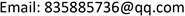Exploring the Relationship between House Prices and Land Prices in Huizhou City Center

—Empirical Analysis Based on Structural Equation Model

Runzhan Guo

School of Urban and Environmental Sciences, Yunnan University of Finance and Economics, Kunming YunnanReceived: Jun. 6th, 2021; accepted: Jul. 8th, 2021; published: Jul. 16th, 2021ABSTRACT

The relationship between urban housing prices and land prices is complex, not only affected by the interweaving of many factors, but also dynamic relationships between them. The tradition of studying the relationship between house prices and land prices, such as Granger causality test and regression analysis, cannot describe the multi-dimensional network relationship between house prices and land prices. In contrast, the structural equation model can handle multiple endogenous latent variables at the same time and is not affected by it. Observing the impact of the collinearity of the indicators provides a new tool for describing the interaction between land prices and housing prices. Starting from the supply and demand transmission mechanism of the housing and land markets, the structure model of house prices and land prices is derived. Taking Huizhou’s central urban areas—Huicheng and Huiyang Districts as examples, the prices of residential land in 2017~2019 and those on sale and for sale in 2020 real estate prices, and then extract explanatory variables from the Fangtianxia webpage, such as public transportation accessibility, prosperous business services and facility convenience, construct a structural equation model of land prices and housing prices, and analyze the structural relationship between the two.

Keywords:House Price, Land Price, Structural Equation Model, Huizhou1. 引言

2. 理论依据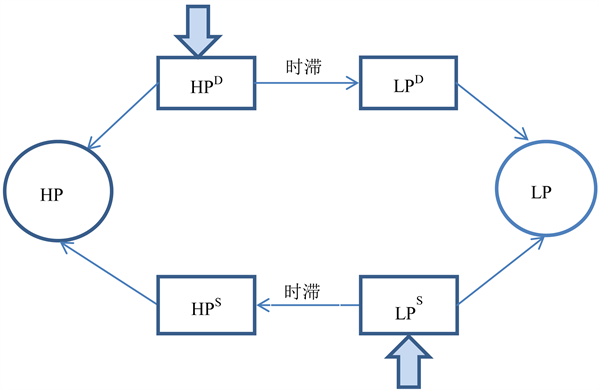Figure 1. Supply and demand chain between house price and land price

3. 模型的推导与构建

3.1. 房价与地价关系的数学推导

“时滞”是房价与地价相互作用的最重要的因素，要研究二者的关系一定要澄清时间起点，如果房价在先，当期房价变动会通过需求传导影响下一期地价；如果地价在先，当期地价会通过供给传导影响下一期房价 。由于房价历史交易信息目前没有公开发布渠道，而相反2017年以来的惠城区和惠阳区的土地出让记录和最新的在售小区信息可以很容易获取，所以采用2017~2019年的地价时间序列数据和2020年房价截面数据作为基础数据集，将土地作为一个房地产运行周期的开端，认为地价先于房价产生，从供给传导的视角出发建立房价与地价关系的模型 。

1) 根据特征价格理论，住房和土地均属于异质性商品，其效用是由一系列属性组合成，如交通条件、基础设施等，因此其需求函数可以表示为一系列属性的函数 ：

$H{P}_{t}^{D}=F\left({x}_{1},{x}_{2},{x}_{3},\cdots ,{x}_{i}\right)$ (1)

$L{P}_{t}^{D}=K\left({y}_{1}+{y}_{2}+\cdots +{y}_{i}\right)$ (2)

2) 构建住房供给函数：

$H{P}_{t}^{S}=H\left(L{P}_{t-1}+C\right)$ (3)

3) 当住房市场与土地市场处于长期均衡时，住房需求价格(HPS)等于住房供给价格(HPD)，土地需求价格(LPS)等于土地供给价格(LPD)，且均衡价格水平(HP与LP)长期保持稳定，即 ：

$HPS=HPD=HP$ (4)

$LPS=LPD=LP$ (5)

$H{P}_{t=1}=H{P}_{t=2}=\cdots =H{P}_{t=n}$ (6)

$L{P}_{t=1}=L{P}_{t=2}=\cdots =L{P}_{t=n}$ (7)

4) 为了简化模型，认为在一个稳定的房地产经济系统中式(4)~(7)是成立的；且仅考虑交通、邻里、设施等同时影响房价和地价的区位因素，即式(1)~式(2)中 ${x}_{i}={y}_{i}$ ；假设与土地成本相比房屋的其他成本C较小可以忽略，函数F、K、H均采用最简单的线性形式。此时式(1)~式(3)可以简化为以下方程组 ：

$\left\{\begin{array}{l}HP={\beta }_{1}{x}_{1}+{\beta }_{2}{x}_{2}+\cdots +{\beta }_{i}{x}_{i}+e\text{\hspace{0.17em}}\text{\hspace{0.17em}}\text{\hspace{0.17em}}\text{\hspace{0.17em}}\text{\hspace{0.17em}}\text{\hspace{0.17em}}\text{\hspace{0.17em}}\text{\hspace{0.17em}}\text{\hspace{0.17em}}\text{\hspace{0.17em}}\text{\hspace{0.17em}}\text{\hspace{0.17em}}\text{\hspace{0.17em}}\text{\hspace{0.17em}}\text{\hspace{0.17em}}\text{\hspace{0.17em}}\text{\hspace{0.17em}}\text{\hspace{0.17em}}\text{\hspace{0.17em}}\text{\hspace{0.17em}}\text{\hspace{0.17em}}\text{\hspace{0.17em}}\text{\hspace{0.17em}}\text{\hspace{0.17em}}\text{\hspace{0.17em}}\text{\hspace{0.17em}}\text{\hspace{0.17em}}\text{\hspace{0.17em}}\text{\hspace{0.17em}}\text{\hspace{0.17em}}\text{\hspace{0.17em}}\text{\hspace{0.17em}}\text{\hspace{0.17em}}\text{\hspace{0.17em}}\text{\hspace{0.17em}}\text{\hspace{0.17em}}\left(\text{A}\right)\\ LP={\lambda }_{1}{x}_{1}+{\lambda }_{2}{x}_{2}+\cdots +{\lambda }_{i}{x}_{i}+\epsilon \text{\hspace{0.17em}}\text{\hspace{0.17em}}\text{\hspace{0.17em}}\text{\hspace{0.17em}}\text{\hspace{0.17em}}\text{\hspace{0.17em}}\text{\hspace{0.17em}}\text{\hspace{0.17em}}\text{\hspace{0.17em}}\text{\hspace{0.17em}}\text{\hspace{0.17em}}\text{\hspace{0.17em}}\text{\hspace{0.17em}}\text{\hspace{0.17em}}\text{\hspace{0.17em}}\text{\hspace{0.17em}}\text{\hspace{0.17em}}\text{\hspace{0.17em}}\text{\hspace{0.17em}}\text{\hspace{0.17em}}\text{\hspace{0.17em}}\text{\hspace{0.17em}}\text{\hspace{0.17em}}\text{\hspace{0.17em}}\text{\hspace{0.17em}}\text{\hspace{0.17em}}\text{\hspace{0.17em}}\text{\hspace{0.17em}}\text{\hspace{0.17em}}\text{\hspace{0.17em}}\text{\hspace{0.17em}}\text{\hspace{0.17em}}\text{\hspace{0.17em}}\text{\hspace{0.17em}}\text{\hspace{0.17em}}\text{\hspace{0.17em}}\text{\hspace{0.17em}}\left(\text{B}\right)\\ HP=\delta LP+\mu \text{\hspace{0.17em}}\text{\hspace{0.17em}}\text{\hspace{0.17em}}\text{\hspace{0.17em}}\text{\hspace{0.17em}}\text{\hspace{0.17em}}\text{\hspace{0.17em}}\text{\hspace{0.17em}}\text{\hspace{0.17em}}\text{\hspace{0.17em}}\text{\hspace{0.17em}}\text{\hspace{0.17em}}\text{\hspace{0.17em}}\text{\hspace{0.17em}}\text{\hspace{0.17em}}\text{\hspace{0.17em}}\text{\hspace{0.17em}}\text{\hspace{0.17em}}\text{\hspace{0.17em}}\text{\hspace{0.17em}}\text{\hspace{0.17em}}\text{\hspace{0.17em}}\text{\hspace{0.17em}}\text{\hspace{0.17em}}\text{\hspace{0.17em}}\text{\hspace{0.17em}}\text{\hspace{0.17em}}\text{\hspace{0.17em}}\text{\hspace{0.17em}}\text{\hspace{0.17em}}\text{\hspace{0.17em}}\text{\hspace{0.17em}}\text{\hspace{0.17em}}\text{\hspace{0.17em}}\text{\hspace{0.17em}}\text{\hspace{0.17em}}\text{\hspace{0.17em}}\text{\hspace{0.17em}}\text{\hspace{0.17em}}\text{\hspace{0.17em}}\text{\hspace{0.17em}}\text{\hspace{0.17em}}\text{\hspace{0.17em}}\text{\hspace{0.17em}}\text{\hspace{0.17em}}\text{\hspace{0.17em}}\text{\hspace{0.17em}}\text{\hspace{0.17em}}\text{\hspace{0.17em}}\text{\hspace{0.17em}}\text{\hspace{0.17em}}\text{\hspace{0.17em}}\text{\hspace{0.17em}}\text{\hspace{0.17em}}\text{ }\text{\hspace{0.17em}}\text{\hspace{0.17em}}\text{\hspace{0.17em}}\text{\hspace{0.17em}}\left( C \right)\end{array}$

3.2. 房价与地价的SEM构建

1) 测量模型构建

$X={\Lambda }_{X}\zeta +\delta$ (8)

$Y={\Lambda }_{Y}\eta +\epsilon$ (9)

SEM中的潜变量包括内生潜变量和外生潜变量，内生潜变量是受到其他潜变量影响的潜变量，相当于因变量；后者则不受其他潜变量影响，仅由观测指标测度的潜变量，相当于自变量。在SEM模型中构地价和房价两个内生潜变量、而区位、交通、基础设施则作为外部要素构成若干的外生潜变量。

2) 结构模型构建

$\eta =B\eta +\Gamma \zeta +\mu$ (10)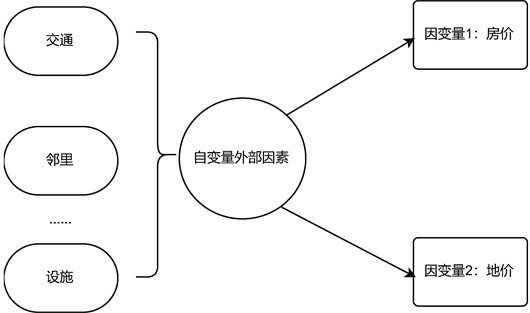Figure 2. SEM diagram of house price and land price

3.3. 参数估计方法

4. 研究区概况

4.1. 惠城区

4.2. 惠阳区

5. 研究方法和思路

1) 通过对地价和房价关系的数学推导，建立二者的函数关系，以此来初步建立关于SEM的房价与地价模型。2) 通过Python编写脚本，对58同城网中的2020年房价数据以及土地资源网、惠州市公共资源交易中心中的2017~2019年地价数据进行爬取，爬取内容包括两套数据：房价、相应坐标；土地成交价、土地出让面积、土地规划建筑面积、相应坐标。对地价数据进行年限修正，并在Python中对爬取的房地价数据进行坐标转换(BD09- > WGS84)。在这基础上，将修正后的地价数据(2017~2020年)，在ArcGIS中进行克里金插值，形成连续的楼面地价表面。然后，通过ArcGIS的点提取，将楼面地价数据提取至房价数据中；最后，收集各个楼盘2000 m范围内的公交站数量、医院数量、学校数量、1000 m内超市和银行的数量，以此作为外生潜变量的观测指标，最后对所有观测变量进行标准化。3) 通过Smart PLS 3软件绘制各潜变量之间的结构模型，并导入标准化的观测变量，绘制测量模型和结构模型，最终得到结构模型的参数估计结果。再对参数估计结果进行信度和效度检验，包括通过区分效度(Average variance extracted, AVE)、复合信度(Composite reliability, CR)和阿尔法系数(Cronbach’s coefficient alpha, CCA)来进行评价。4) 对估计和检验结果进行分析，得出房价与地价之间的关系。对应的技术路线图，如图3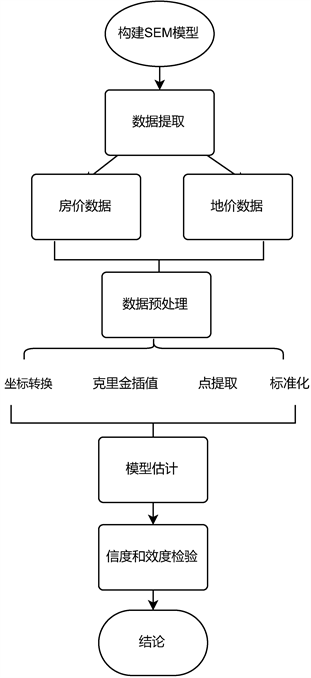6. 数据来源和数据处理

6.1. 样本提取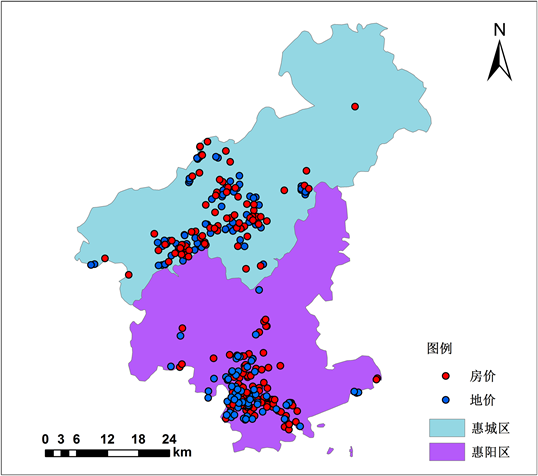Figure 4. Distribution of house prices and land prices

6.2. 数据预处理

1) 根据土地成交价和容积率计算出楼面地价FP。

2) 选择惠州市住宅用地的地价指数作为修正指数对出让期进行修正，剔除样本时间跨度内的通货膨胀、经济增长、房地产市场波动等时间趋势。

3) 将2017~2020年(惠城区样本量N = 85、惠阳区样本量N = 65)地价样本数据分为两组，在ArGIS中通过Spatial Statistics Tools中的空间自相关分析，分析研究区楼面地价的自相关性，通过分析发现惠城区和惠阳区中Z-score > 2.58，表明以99%的置信水平支持楼面地价存在空间自相关这一假设，满足空间插值的前提条件，则再利用Geostatistical Analyst Tools中的普通克里金插值法分别进行空间插值，形成连续的楼面地价表面，插值结果如下图5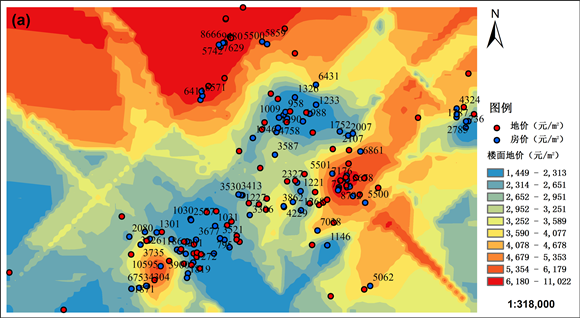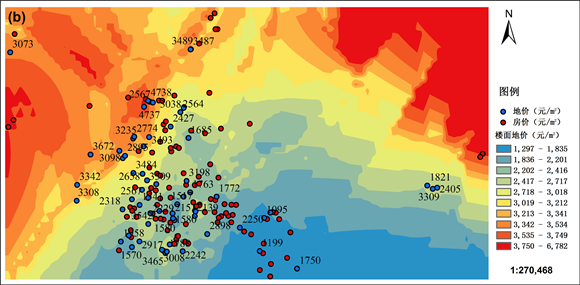Figure 5. Interpolation map of floor price of residential land in Huizhou from 2017 to 2019 ((a) Huicheng District; (b) Huiyang District)

7. 模型估计与效果分析

7.1. 模型设计Table 1. SEM Structure of endogenous latent variables

7.2. 模型估计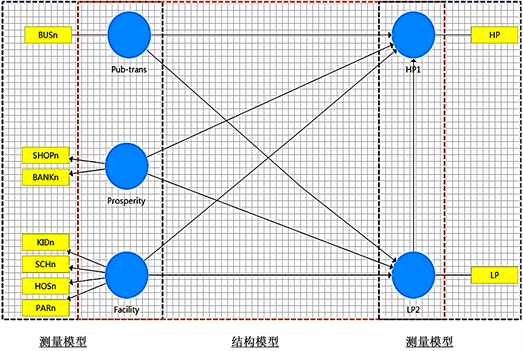Figure 6. SEM structure of model 1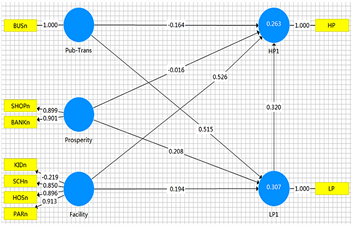(a) Model1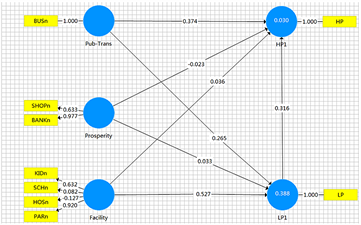(b) Model1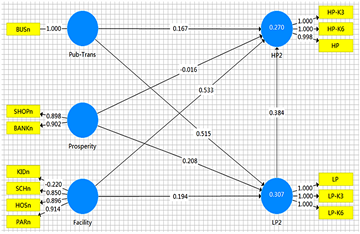(c) Model2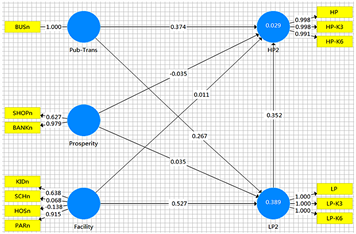(d) Model2

Figure 7. SEM Parameter estimation results ((a), (c) on behalf of Huicheng District, (b), (d) on behalf of Huiyang District)

7.3. 测量模型效果评测

7.4. 结构模型效果评测

R2表示的是构建模型的拟合程度和对外生潜变量的解释程度，当R2 = 0.33左右表示具有中度解释能力，当R2 > 0.67表示解释能力较好。而从表6可以看出，所构建的模型普遍对地价的解释能力较强，基本达到了0.5，但是对房价的解释能力为中等。这表明所选取的外生潜变量对地价的拟合度或是解释能力比房价更高，同时发现，由于模型2考虑了房价和地价的空间相关性，所以模型2拟合情况有所改善，这表明无论房价和地价，其在空间中并不是孤立存在的，在一定的距离范围内是存在相互影响，而这主要是因为其所处的区位存在相似性和可比性。

8. 结果分析

8.1. 房价与地价的结构关系Figure 8. Relationship between land price and house price

8.2. 地价对房价的影响程度

9. 结论和不足

9.1. 结论

1) 土地市场与住房市场之间通过供给和需求紧密联系，当期房价的上涨可以通过需求传导拉动下一期地价，当期地价的上涨也可以通过供给传导推升下一期房价。实证分析的结果表明，惠州市惠城区和惠阳区2020年房价受2017~2019年住宅用地的出让价格显著影响，影响方向为正，这验证了往期地价对当期房价存在推动作用。并且当综合考虑了房价和地价的空间相关性后，这一影响的估计值变大，根据SEM估计结果，推导出惠州市中心城区地价对房价的影响的路径系数大致为0.3~0.38。

2) 区位因子对地价和房价的作用多样化，有的对地价显著，有的同时对地价和房价具有显著影响。而这一现象的原因是由于部分区位因素主要是通过供给端影响房价，部分因素是通过需求端影响房价。根据SEM估计结果，在惠城区房地产市场中，商服繁华度更多地是通过影响土地需求函数来影响地价，从而通过影响成本来影响房价。而公共交通可达性和设施便利度则可以直接影响住房需求函数，来影响房价。

9.2. 不足

Exploring the Relationship between House Prices and Land Prices in Huizhou City Center[J]. 城镇化与集约用地, 2021, 09(03): 29-44. https://doi.org/10.12677/ULU.2021.93005

1. 1. 陈日清. 我国房价与地价的非线性均衡关系研究[J]. 建筑经济, 2015, 36(11): 74-77.

2. 2. 温海珍, 吕雪梦, 张凌. 房价与地价的内生性及其互动影响——基于联立方程模型的实证分析[J]. 财贸经济, 2010(2): 124-129.

3. 3. 孙波, 李惠. 地价推动下的房价上涨逻辑与影响[J]. 商业研究, 2010(5): 160-166.

4. 4. 严金海. 中国的房价与地价: 理论、实证和政策分析[J]. 数量经济技术经济研究, 2006, 23(1): 17-26.

5. 5. 武文杰, 刘志林, 张文忠. 基于结构方程模型的北京居住用地价格影响因素评价[J]. 地理学报, 2010, 65(6): 676-684.

6. 6. 孟勉, 李文斌. Hedonic和结构方程模型下的北京别墅价格分析[J]. 经济地理, 2006(S1): 166-171.

7. 7. 朱明仓, 吴合镇, 辜寄蓉. 重庆市房价地价动态关系模型构建[J]. 成都理工大学学报: 自然科学版, 2008, 35(5): 572-579.

8. 8. 辜寄蓉, 朱明仓, 吴合镇. 重庆市房价与地价影响因素实证研究[J]. 测绘与空间地理信息, 2009, 32(4): 1-4.

9. 9. 甘霖, 冯长春, 王乾. 北京市房价与地价的动态关系——基于结构方程模型的实证分析[J]. 地理研究, 2016, 35(10): 35-49.

10. 10. 周彬, 杜两省. “土地财政”与房地产价格上涨: 理论分析和实证研究[J]. 财贸经济, 2010(8): 109-116.

11. 11. 郭辉. 现阶段我国房价上涨的地价因素分析[J]. 南方经济, 2005(8): 31-33.

12. 12. 张继道, 卢新海, 李学文. 地价与房价的因果关系——基于武汉市的实证研究[J]. 中国房地产, 2011(16): 20-27.